# 外汇EA编写教程：图形界面 X: 渲染表格的新功能 (集成编译 9)

### 概论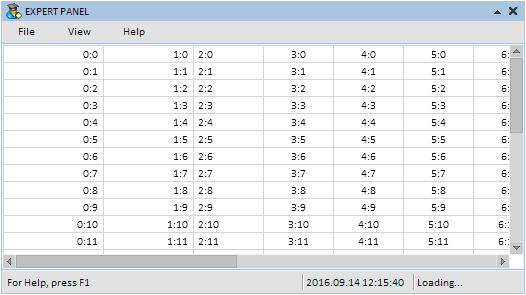• 格式化斑马样式
• 选择表格行, 再次单击时弃选
• 添加了列标题, 可在鼠标悬浮和点击时改变颜色
• 当单元格空间不足时, 则依照文本自动调整列宽
• 能够通过拖动其边框来改变每列的标题宽度

### 格式化斑马样式

```//+------------------------------------------------------------------+
//| 创建渲染表格的类                                                    |
//+------------------------------------------------------------------+
class CCanvasTable : public CElement
{
private:
//--- 格式化斑马样式模式
color             m_is_zebra_format_rows;
//---
public:
//--- 格式化斑马样式
void              IsZebraFormatRows(const color clr)   { m_is_zebra_format_rows=clr;      }
};

```

```class CCanvasTable : public CElement
{
public:
//--- 绘制表格行的背景
void              DrawRows(void);
};
//+------------------------------------------------------------------+
//| 绘制表格行的背景                                                    |
//+------------------------------------------------------------------+
void CCanvasTable::DrawRows(void)
{
//--- 如果禁用格式化斑马样式模式
if(m_is_zebra_format_rows==clrNONE)
{
//--- 用一种颜色填充画板
m_table.Erase(::ColorToARGB(m_cell_color));
return;
}
//--- 标题坐标
int x1=0,x2=m_table_x_size;
int y1=0,y2=0;
//--- 格式化斑马样式
for(int r=0; r<m_rows_total; r++)
{
//--- 计算坐标
y1=(r*m_cell_y_size)-r;
y2=y1+m_cell_y_size;
//--- 行颜色
uint clr=::ColorToARGB((r%2!=0)? m_is_zebra_format_rows : m_cell_color);
//--- 绘制行背景
m_table.FillRectangle(x1,y1,x2,y2,clr);
}
}

```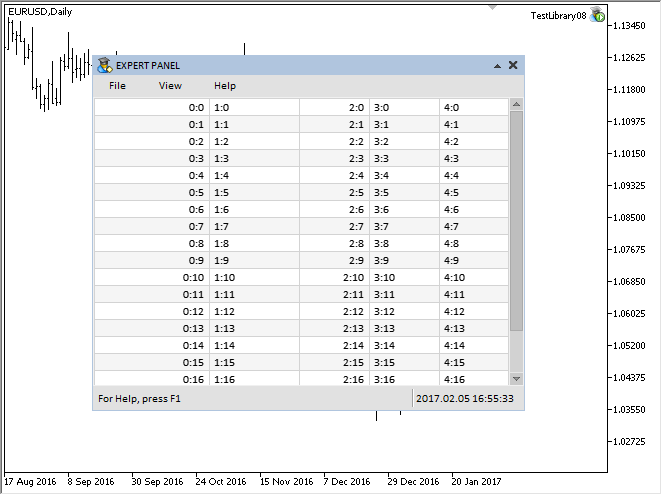### 选择和弃选表格行

• 所选行的背景和文本的颜色
• 索引和文本
```class CCanvasTable : public CElement
{
private:
//--- 颜色用于 (1) 背景 (2) 所选行文本
color             m_selected_row_color;
color             m_selected_row_text_color;
//--- (1) 索引 (2) 所选行文本
int               m_selected_item;
string            m_selected_item_text;
//---
public:
//--- 返回 (1) 索引 (2) 表格中所选行文本
int               SelectedItem(void)             const { return(m_selected_item);         }
string            SelectedItemText(void)         const { return(m_selected_item_text);    }
//---
private:
//--- 绘制表格行的背景
void              DrawRows(void);
};

```

```class CCanvasTable : public CElement
{
private:
//--- 可选行模式
bool              m_selectable_row;
//---
public:
//--- 行选择
void              SelectableRow(const bool flag)       { m_selectable_row=flag;           }
};

```

```class CCanvasTable : public CElement
{
private:
//--- 绘制所选行
void              DrawSelectedRow(void);
};
//+------------------------------------------------------------------+
//| 绘制所选行                                                         |
//+------------------------------------------------------------------+
void CCanvasTable::DrawSelectedRow(void)
{
//--- 设置初始坐标以便检查条件
int y_offset=(m_selected_item*m_cell_y_size)-m_selected_item;
//--- 坐标
int x1=0,x2=0,y1=0,y2=0;
//---
x1=0;
y1=y_offset;
x2=m_table_x_size;
y2=y_offset+m_cell_y_size-1;
//--- 绘制填充矩形
m_table.FillRectangle(x1,y1,x2,y2,::ColorToARGB(m_selected_row_color));
}

```

```class CCanvasTable : public CElement
{
private:
//--- 返回单元格文本的颜色
uint              TextColor(const int row_index);
};
//+------------------------------------------------------------------+
//| 返回单元格文本的颜色                                                 |
//+------------------------------------------------------------------+
uint CCanvasTable::TextColor(const int row_index)
{
uint clr=::ColorToARGB((row_index==m_selected_item)? m_selected_row_text_color : m_cell_text_color);
//--- 返回标题颜色
return(clr);
}

```

• 行选择模式被禁用;
• 滚动条已激活;
• 未在表格上点击。

• ON_CLICK_LIST_ITEM 事件的标识符
• 控件的标识符
• 所选行的索引
• 所选行的文本。
```class CCanvasTable : public CElement
{
private:
//--- 在元素上点按处理
bool              OnClickTable(const string clicked_object);
};
//+------------------------------------------------------------------+
//| 点击控件处理                                                       |
//+------------------------------------------------------------------+
bool CCanvasTable::OnClickTable(const string clicked_object)
{
//--- 如果禁用选择行模式, 离开
if(!m_selectable_row)
return(false);
//--- 如果滚动条激活, 离开
if(m_scrollv.ScrollState() || m_scrollh.ScrollState())
return(false);
//--- 如果对象名不同, 离开
if(m_table.Name()!=clicked_object)
return(false);
//--- 获取 X 和 Y 轴的偏移量
int xoffset=(int)m_table.GetInteger(OBJPROP_XOFFSET);
int yoffset=(int)m_table.GetInteger(OBJPROP_YOFFSET);
//--- 确定鼠标光标下方的文本编辑框坐标
int y=m_mouse.Y()-m_table.Y()+yoffset;
//--- 确定点击的行
for(int r=0; r<m_rows_total; r++)
{
//--- 设置初始坐标以便检查条件
int y_offset=(r*m_cell_y_size)-r;
//--- 沿 Y 轴检查状态
bool y_pos_check=(y>=y_offset && y<y_offset+m_cell_y_size);
//--- 如果点击并非此行, 则转至下一个
if(!y_pos_check)
continue;
//--- 如果点击已选行, 取消选择
if(r==m_selected_item)
{
m_selected_item      =WRONG_VALUE;
m_selected_item_text ="";
break;
}
//--- 保存行索引
m_selected_item      =r;
m_selected_item_text =m_vcolumns.m_vrows[r];
break;
}
//--- 表格重绘
DrawTable();
//--- 发送有关消息
::EventChartCustom(m_chart_id,ON_CLICK_LIST_ITEM,CElementBase::Id(),m_selected_item,m_selected_item_text);
return(true);
}

```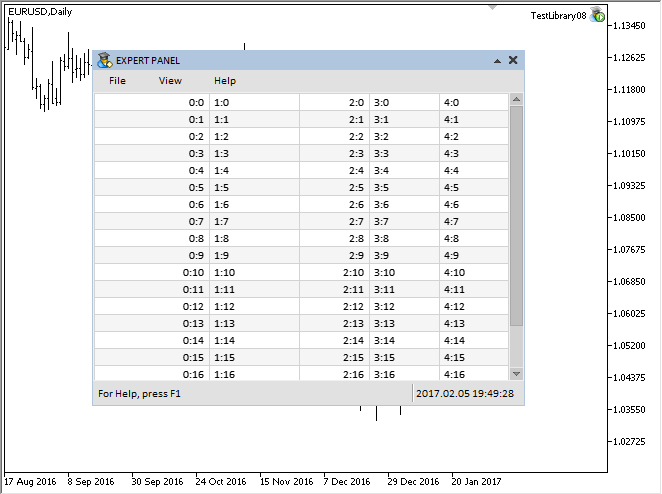### 列标题

```class CCanvasTable : public CElement
{
private:
//--- 创建表格的对象
//---
private:
};

```

• 表头的显示模式。
• 标题的大小 (高度)。
• 不同状态下的标题背景颜色。
• 标题文本颜色。

```class CCanvasTable : public CElement
{
private:
//--- 表头的显示模式
//--- 标题的大小 (高度)
//--- 不同状态下的标题 (背景) 颜色
//--- 标题文本颜色
//---
public:
//--- (1) 标题显示模式, (2) 标题高度
//--- (1) 背景 (2) 标题文本颜色
};

```

```class CCanvasTable : public CElement
{
private:
//--- 标题文本
//---
public:
//--- 设置指定标题的文本
void              SetHeaderText(const int column_index,const string value);
};
//+------------------------------------------------------------------+
//| 在指定索引处填充标题数组                                              |
//+------------------------------------------------------------------+
void CCanvasTable::SetHeaderText(const uint column_index,const string value)
{
//--- 检查列超界
uint csize=::ArraySize(m_vcolumns);
if(csize<1 || column_index>=csize)
return;
//--- 将数值保存到数组中
}

```

```class CCanvasTable : public CElement
{
private:
//--- 返回指定列中的文本对齐模式
uint              TextAlign(const int column_index,const uint anchor);
};
//+------------------------------------------------------------------+
//| 返回指定列中的文本对齐模式                                            |
//+------------------------------------------------------------------+
uint CCanvasTable::TextAlign(const int column_index,const uint anchor)
{
uint text_align=0;
//--- 当前列的文本对齐
switch(m_vcolumns[column_index].m_text_align)
{
case ALIGN_CENTER :
text_align=TA_CENTER|anchor;
break;
case ALIGN_RIGHT :
text_align=TA_RIGHT|anchor;
break;
case ALIGN_LEFT :
text_align=TA_LEFT|anchor;
break;
}
//--- 返回对齐类型
return(text_align);
}

```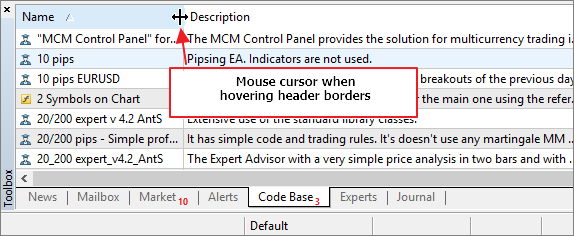```//+------------------------------------------------------------------+
//| 指针类型的枚举                                                      |
//+------------------------------------------------------------------+
enum ENUM_MOUSE_POINTER
{
MP_CUSTOM            =0,
MP_X_RESIZE          =1,
MP_Y_RESIZE          =2,
MP_XY1_RESIZE        =3,
MP_XY2_RESIZE        =4,
MP_X_RESIZE_RELATIVE =5,
MP_Y_RESIZE_RELATIVE =6,
MP_X_SCROLL          =7,
MP_Y_SCROLL          =8,
MP_TEXT_SELECT       =9
};

```

```//+------------------------------------------------------------------+
//|                                                      Pointer.mqh |
//|                                 版权所有 2015, MetaQuotes 软件公司  |
//|                                              https://www.mql5.com |
//+------------------------------------------------------------------+
//--- 资源
#resource "//Images//EasyAndFastGUI//Controls//pointer_x_rs_rel.bmp"
#resource "//Images//EasyAndFastGUI//Controls//pointer_y_rs_rel.bmp"
//+------------------------------------------------------------------+
//| 创建鼠标光标的类                                                    |
//+------------------------------------------------------------------+
class CPointer : public CElement
{
private:
//--- 设置鼠标光标的图像
void              SetPointerBmp(void);
};
//+------------------------------------------------------------------+
//| 基于光标类型设置光标图标                                              |
//+------------------------------------------------------------------+
void CPointer::SetPointerBmp(void)
{
switch(m_type)
{
...
case MP_X_RESIZE_RELATIVE :
m_file_on  ="Images//EasyAndFastGUI//Controls//pointer_x_rs_rel.bmp";
m_file_off ="Images//EasyAndFastGUI//Controls//pointer_x_rs_rel.bmp";
break;
case MP_Y_RESIZE_RELATIVE :
m_file_on  ="Images//EasyAndFastGUI//Controls//pointer_y_rs_rel.bmp";
m_file_off ="Images//EasyAndFastGUI//Controls//pointer_y_rs_rel.bmp";
break;
...
}
//--- 如果指定为自定义类型 (MP_CUSTOM)
if(m_file_on=="" || m_file_off=="")
::Print(__FUNCTION__," > 必须为光标设置两个图像");
}

```

• 确定拖动标题边框的时刻
• 为了确定鼠标光标从一个标题区域移动到另一个标题区域的时刻。这需要节省资源, 因此只有当相邻区域的边界交错时才会重新绘制标题。
```class CCanvasTable : public CElement
{
private:
//--- 确定鼠标光标从一个标题转换到另一个标题的时刻
//--- 拖动标题边框以改变列宽的状态
int               m_column_resize_control;
};

```

```class CCanvasTable : public CElement
{
private:
//--- 返回当前的标题背景色
};
//+------------------------------------------------------------------+
//| 返回当前的标题背景色                                                 |
//+------------------------------------------------------------------+
{
uint clr=clrNONE;
//--- 如果无焦点
else
{
//--- 如果鼠标左键按下且未处于列宽改变进程
bool condition=(m_mouse.LeftButtonState() && m_column_resize_control==WRONG_VALUE);
}
//--- 返回标题颜色
return(::ColorToARGB(clr));
}

```

CCanvasTable::DrawHeaders() 方法的代码表述如下。此处, 如果鼠标光标不在标题区域, 则 整个画板将以指定的颜色填充。如果焦点在标题上, 那么有必要确定它们当中的哪一个拥有焦点。为此, 需要确定鼠标光标的相对坐标, 并在计算标题坐标的循环中检查每个标题是否处于焦点。另外, 在此还要考虑改变列宽模式。在计算此模式中使用 附加偏移量如果找到焦点, 则必须保存列索引。

```class CCanvasTable : public CElement
{
private:
//--- 自分隔线边界的偏移, 按照更改列宽模式显示鼠标指针
int               m_sep_x_offset;
//---
private:
//--- 绘制标题
};
//+------------------------------------------------------------------+
//| 绘制标题背景                                                       |
//+------------------------------------------------------------------+
{
//--- 如果非焦点, 重置标题颜色
{
return;
}
//--- 检查焦点是否在标题上
//--- 鼠标光标坐标
int x=0;
//--- 坐标
//--- 得到鼠标光标的相对坐标
if(::CheckPointer(m_mouse)!=POINTER_INVALID)
{
//--- 获取 X 轴偏移量
//--- 确定鼠标光标坐标
}
//--- 清除标题背景
//--- 考虑到更改列宽模式偏移
int sep_x_offset=(m_column_resize_mode)? m_sep_x_offset : 0;
//--- 绘制标题背景
for(int i=0; i<m_columns_total; i++)
{
//--- 计算坐标
x2+=m_vcolumns[i].m_width;
//--- 检查焦点
if(is_header_focus=x>x1+((i!=0)? sep_x_offset : 0) && x<=x2+sep_x_offset)
//--- 绘制标题背景
//--- 计算下一标题偏移
x1+=m_vcolumns[i].m_width;
}
}

```

```class CCanvasTable : public CElement
{
private:
//--- 绘制表格标题网格
};
//+------------------------------------------------------------------+
//| 绘制表格标题网格                                                    |
//+------------------------------------------------------------------+
{
//--- 网格颜色
uint clr=::ColorToARGB(m_grid_color);
//--- 坐标
int x1=0,x2=0,y1=0,y2=0;
x2=m_table_x_size-1;
//--- 绘制边框
//--- 分割线
x2=x1=m_vcolumns.m_width;
for(int i=1; i<m_columns_total; i++)
{
x2=x1+=m_vcolumns[i].m_width;
}
}

```

```class CCanvasTable : public CElement
{
private:
//--- 绘制表格标题文本
};
//+------------------------------------------------------------------+
//| 绘制表格标题文本                                                    |
//+------------------------------------------------------------------+
{
//--- 计算坐标和偏移
int column_offset =0;
uint text_align   =0;
//--- 文本颜色
//--- 字体属性
//---绘制文本
for(int c=0; c<m_columns_total; c++)
{
//--- 获取文本的 X 坐标
x=TextX(c,column_offset);
//--- 获取文本的对齐模式
text_align=TextAlign(c,TA_VCENTER);
//--- 绘制列名称
}
}

```

```class CCanvasTable : public CElement
{
private:
//--- 绘制表格标题
};
//+------------------------------------------------------------------+
//| 绘制表格标题                                                       |
//+------------------------------------------------------------------+
{
//--- 如果禁用标题, 离开
return;
//--- 绘制标题
//--- 绘制网格
//--- 绘制标题文本
}

```

• 如果标题显示模式被禁用
• 或者如果更改列宽的过程已经开始。

```class CCanvasTable : public CElement
{
private:
//--- 检查标题上的焦点
};
//+------------------------------------------------------------------+
//| 检查标题上的焦点                                                    |
//+------------------------------------------------------------------+
{
//--- 如果 (1) 标题被禁用 (2) 列宽变更已开始, 离开
return;
//--- 标题坐标
int x1=0,x2=0;
//--- 获取 X 轴偏移量
//--- 得到鼠标光标的相对坐标
//--- 考虑到更改列宽模式偏移
int sep_x_offset=(m_column_resize_mode)? m_sep_x_offset : 0;
//--- 搜索焦点
for(int i=0; i<m_columns_total; i++)
{
//--- 计算右坐标
x2+=m_vcolumns[i].m_width;
//--- 如果标题焦点改变
{
break;
}
//--- 计算左坐标
x1+=m_vcolumns[i].m_width;
}
}

```

```class CCanvasTable : public CElement
{
private:
//--- 改变标题颜色
};
//+------------------------------------------------------------------+
//| 改变标题颜色                                                       |
//+------------------------------------------------------------------+
{
//--- 如果禁用标题, 离开
return;
//--- 如果光标已激活
if(m_column_resize.IsVisible() && m_mouse.LeftButtonState())
{
//--- 保存拖拽列的索引
if(m_column_resize_control==WRONG_VALUE)
//---
return;
}
//--- 非焦点
{
//--- 如果尚未指出不在焦点
{
//--- 重置焦点
//--- 改变颜色
}
}
//--- 若在焦点
else
{
//--- 检查标题的焦点
//--- 如果无焦点
{
//--- 改变颜色
}
}
}

```

```class CCanvasTable : public CElement
{
private:
//--- 检查标题边框上的焦点以更改其宽度
void              CheckColumnResizeFocus(void);
};
//+------------------------------------------------------------------+
//| 检查标题边框上的焦点以更改其宽度                                       |
//+------------------------------------------------------------------+
void CCanvasTable::CheckColumnResizeFocus(void)
{
//--- 如果禁用更改列宽模式, 离开
if(!m_column_resize_mode)
return;
//--- 如果开始更改列宽, 离开
if(m_column_resize_control!=WRONG_VALUE)
{
//--- 更新光标坐标并令其可见
m_column_resize.Moving(m_mouse.X(),m_mouse.Y());
return;
}
//--- 检查标题边框上的焦点
bool is_focus=false;
//--- 如果鼠标光标位于标题区域
{
//--- 标题坐标
int x1=0,x2=0;
//--- 获取 X 轴偏移量
//--- 得到鼠标光标的相对坐标
//--- 搜索焦点
for(int i=0; i<m_columns_total; i++)
{
//--- 计算坐标
x1=x2+=m_vcolumns[i].m_width;
//--- 验证焦点
if(is_focus=x>x1-m_sep_x_offset && x<=x2+m_sep_x_offset)
break;
}
//--- 如果这是焦点
if(is_focus)
{
//--- 更新光标坐标并令其可见
m_column_resize.Moving(m_mouse.X(),m_mouse.Y());
//--- 显示光标
m_column_resize.Show();
return;
}
}
//--- 如果非焦点, 隐藏指针
m_column_resize.Hide();
}

```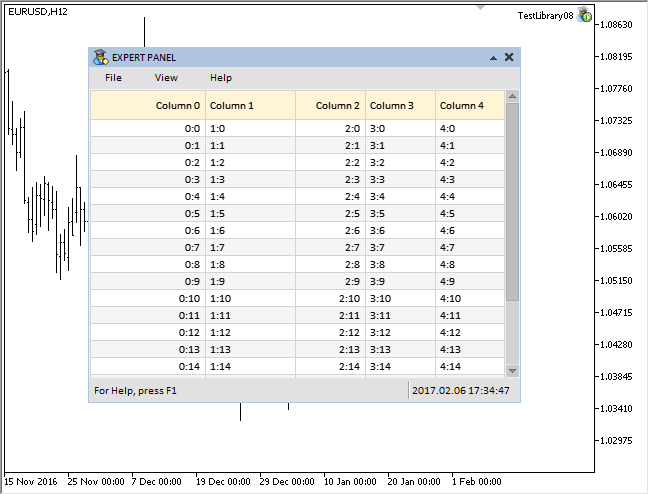### 相对于列宽调整字符串长度

```class CCanvasTable : public CElement
{
private:
//--- 表格数值和属性的数组
struct CTOptions
{
string            m_vrows[];
string            m_text[];
int               m_width;
ENUM_ALIGN_MODE   m_text_align;
};
CTOptions         m_vcolumns[];
};

```

CCanvasTable::CorrectingText() 方法将负责调整 标题和表格单元两者内的 字符串长度。在识别操作的文本之后, 获取其宽度。接下来, 检查字符串的全文是否适合单元格, 同时考虑单元格边缘的所有偏移量。如果它适合, 将之保存在 m_text[] 数组里并离开方法。在当前版本中, 仅保存调整过的单元格文本, 但不针对标题。

1)获取字符串长度。

2)之后在循环里从最后一个字符开始遍历所有字符, 删除最后一个字符并将修剪后的文本保存在临时变量中。

4)只要有字符剩下, 获取包含省略号在内的结果字符串宽度。

5)检查依次形成的字符串是否适合表格单元, 同时考虑自单元格边缘指定的偏移。

6)如果字符串合适, 则将其保存到方法的局部变量中并停止循环。

7)此后, 将调整后的字符串保存到 m_text[] 数组中, 并从方法返回。

```class CCanvasTable : public CElement
{
private:
//--- 返回依据列宽调整的文本
string            CorrectingText(const int column_index,const int row_index,const bool headers=false);
};
//+------------------------------------------------------------------+
//| 返回依据列宽调整的文本                                               |
//+------------------------------------------------------------------+
string CCanvasTable::CorrectingText(const int column_index,const int row_index,const bool headers=false)
{
//--- 获取当前文本
//--- 自单元格边缘在 X 轴上的偏移
int x_offset=m_text_x_offset*2;
//--- 获取画板对象的指针
//--- 获取文本宽度
int full_text_width=obj.TextWidth(corrected_text);
//--- 如果它适合单元格, 将调整后的文本保存在单独的数组中并返回
if(full_text_width<=m_vcolumns[column_index].m_width-x_offset)
{
//--- 如果这些不是标题, 保存调整后的文本
m_vcolumns[column_index].m_text[row_index]=corrected_text;
//---
return(corrected_text);
}
//--- 如果文本不适合单元格, 则需要调整文本 (修剪过多字符并添加省略号)
else
{
//--- 用来操纵字符串
string temp_text="";
//--- 获取字符串长度
int total=::StringLen(corrected_text);
//--- 从字符串中逐个删除字符, 直到所需文本宽度
for(int i=total-1; i>=0; i--)
{
//--- 删除一个字符
temp_text=::StringSubstr(corrected_text,0,i);
//--- 如果什么都没剩下, 保留空字符串
if(temp_text=="")
{
corrected_text="";
break;
}
//--- 在检查之前添加省略号
int text_width=obj.TextWidth(temp_text+"...");
//--- 如果适合单元格
if(text_width<m_vcolumns[column_index].m_width-x_offset)
{
//--- 保存文本并停止循环
corrected_text=temp_text+"...";
break;
}
}
}
//--- 如果这些不是标题, 保存调整后的文本
m_vcolumns[column_index].m_text[row_index]=corrected_text;
//--- 返回调整后的文字
return(corrected_text);
}

```

CCanvasTable::Text() 方法将确定是否需要调整指定列的文本, 或者发送以前调整的版本即可足矣。其代码如下所见:

```class CCanvasTable : public CElement
{
private:
//--- 返回文本
string            Text(const int column_index,const int row_index);
};
//+------------------------------------------------------------------+
//| 返回文本                                                           |
//+------------------------------------------------------------------+
string CCanvasTable::Text(const int column_index,const int row_index)
{
string text="";
//--- 如果不是更改列宽模式, 调整文本
if(m_column_resize_control==WRONG_VALUE)
text=CorrectingText(column_index,row_index);
//--- 如果处于更改列宽度模式下, 则...
else
{
//--- ...仅宽度改变的列需要调整文本
if(column_index==m_column_resize_control)
text=CorrectingText(column_index,row_index);
//--- 对于所有其它, 使用以前调整的文字
else
text=m_vcolumns[column_index].m_text[row_index];
}
//--- 返回文本
return(text);
}

```

```class CCanvasTable : public CElement
{
private:
//--- 列的最小宽度
int               m_min_column_width;
//---
private:
//--- 更改拖拽列的宽度
void              ChangeColumnWidth(void);
};
//+------------------------------------------------------------------+
//| 构造器                                                            |
//+------------------------------------------------------------------+
CCanvasTable::CCanvasTable(void) : m_min_column_width(30)
{
...
}
//+------------------------------------------------------------------+
//| 更改拖拽列的宽度                                                    |
//+------------------------------------------------------------------+
void CCanvasTable::ChangeColumnWidth(void)
{
//--- 如果禁用标题, 离开
return;
//--- 检查标题边界的焦点
CheckColumnResizeFocus();
//--- 辅助变量
static int x_fixed    =0;
static int prev_width =0;
//--- 如果完成, 重置该值
if(m_column_resize_control==WRONG_VALUE)
{
x_fixed    =0;
prev_width =0;
return;
}
//--- 获取 X 轴偏移量
//--- 得到鼠标光标的相对坐标
//--- 如果更改列宽的过程刚刚开始
if(x_fixed<1)
{
//--- 保存当前列的 X 坐标和宽度
x_fixed    =x;
prev_width =m_vcolumns[m_column_resize_control].m_width;
}
//--- 计算列的新宽度
int new_width=prev_width+(x-x_fixed);
//--- 如果小于指定的极限, 保持不变
if(new_width<m_min_column_width)
return;
//--- 保存列的新宽度
m_vcolumns[m_column_resize_control].m_width=new_width;
//--- 计算表格大小
CalculateTableSize();
//--- 调整表的大小
ChangeTableSize();
//--- 表格重绘
DrawTable();
}

```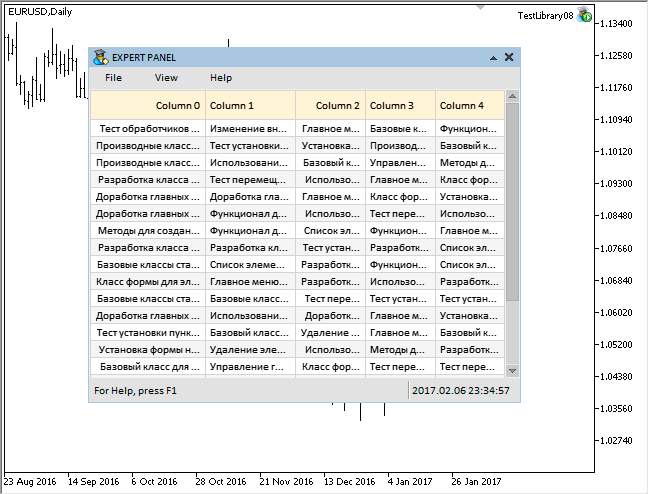### 事件处理

```//+------------------------------------------------------------------+
//| 事件处理器                                                         |
//+------------------------------------------------------------------+
void CCanvasTable::OnEvent(const int id,const long &lparam,const double &dparam,const string &sparam)
{
//--- 处理光标移动事件
if(id==CHARTEVENT_MOUSE_MOVE)
{
//--- 如果控件被隐藏, 离开
if(!CElementBase::IsVisible())
return;
//--- 如果子窗口的数字不匹配, 离开
if(!CElementBase::CheckSubwindowNumber())
return;
//--- 检查焦点覆盖在元素上
CElementBase::CheckMouseFocus();
//--- 如果滚动条处于激活状态
if(m_scrollv.ScrollBarControl() || m_scrollh.ScrollBarControl())
{
ShiftTable();
return;
}
//--- 更改对象颜色
ChangeObjectsColor();
//--- 更改拖拽列的宽度
ChangeColumnWidth();
return;
}
...
}

```

```//+------------------------------------------------------------------+
//|                                                      Defines.mqh |
//|                                 版权所有 2015, MetaQuotes 软件公司|
//|                                              https://www.mql5.com |
//+------------------------------------------------------------------+
#define ON_CHANGE_MOUSE_LEFT_BUTTON (33) // 改变鼠标左键的状态

```

```//+------------------------------------------------------------------+
//| 获取鼠标参数的类                                                    |
//+------------------------------------------------------------------+
class CMouse
{
private:
//--- 检查鼠标左键的状态变化
bool              CheckChangeLeftButtonState(const string mouse_state);
};
//+------------------------------------------------------------------+
//| 处理移动鼠标光标的事件                                               |
//+------------------------------------------------------------------+
void CMouse::OnEvent(const int id,const long &lparam,const double &dparam,const string &sparam)
{
//--- 处理光标移动事件
if(id==CHARTEVENT_MOUSE_MOVE)
{
//--- 坐标和鼠标左键的状态
m_x                 =(int)lparam;
m_y                 =(int)dparam;
m_left_button_state =CheckChangeLeftButtonState(sparam);
...
}
}
//+------------------------------------------------------------------+
//| 检查鼠标左键的状态变化                                               |
//+------------------------------------------------------------------+
bool CMouse::CheckChangeLeftButtonState(const string mouse_state)
{
bool left_button_state=(bool)int(mouse_state);
//--- 发送关于鼠标左键状态更改的消息
if(m_left_button_state!=left_button_state)
::EventChartCustom(m_chart.ChartId(),ON_CHANGE_MOUSE_LEFT_BUTTON,0,0.0,"");
//---
return(left_button_state);
}

```

•  将类中的某些字段归零;
•  调整滚动条;
•  重绘表格
```//+------------------------------------------------------------------+
//| 事件处理器                                                         |
//+------------------------------------------------------------------+
void CCanvasTable::OnEvent(const int id,const long &lparam,const double &dparam,const string &sparam)
{
//--- 更改鼠标左键的状态
if(id==CHARTEVENT_CUSTOM+ON_CHANGE_MOUSE_LEFT_BUTTON)
{
//--- 如果禁用标题, 离开
return;
//--- 如果鼠标左键释放
if(!m_mouse.LeftButtonState())
{
//--- 重置宽度更改模式
m_column_resize_control=WRONG_VALUE;
//--- 隐藏光标
m_column_resize.Hide();
//--- 考虑最近变化来调整滚动条
HorizontalScrolling(m_scrollh.CurrentPos());
}
//--- 重置标题上最后一个焦点的索引
//--- 更改对象颜色
ChangeObjectsColor();
}
}

```

### 结论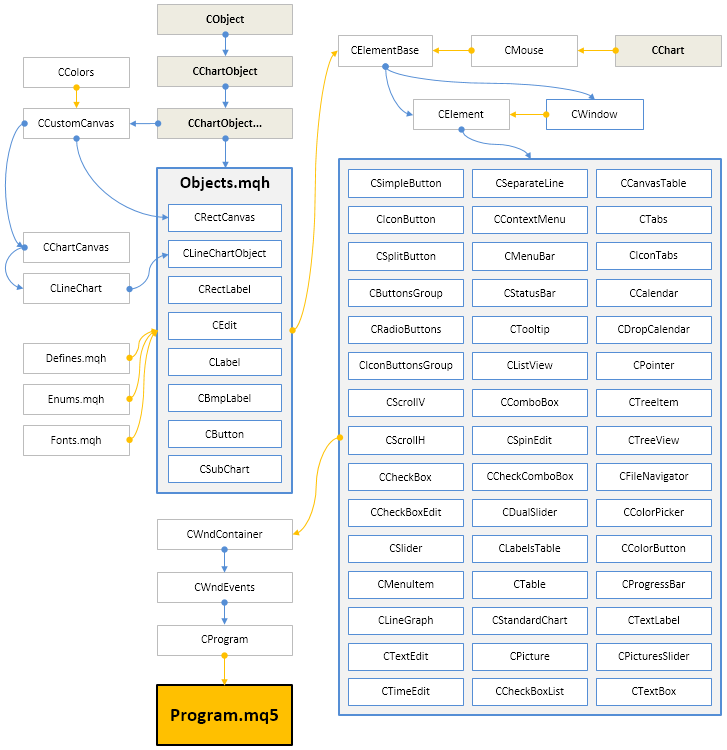MyFxtop迈投-靠谱的外汇跟单社区，免费跟随高手做交易！